# Image Effects with PHP and GD

 This tutorial covers some PHP GD scripts that emulate imagefilter effects without using a filter. These scripts have been collected over the years from the internet, and some are no longer on line. Some come from the new site of Hudzilla but taken from the old site, which is no longer on line. Some of them also have complicated scripting, and functions not covered in these tutorials. You can still use them by adding your url and other parameters needed for the script. Good way to learn. ORIGINAL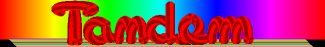BRIGHTNESS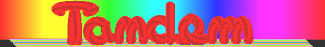Factor 50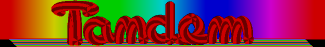Factor -50 Right click, select all, ctrl+c to copy. PIXELATE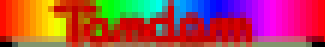Blocksize 6 Right click, select all, ctrl+c to copy. = \$imagex) { \$colours[] = \$thiscol; continue; } if (\$l < 0) { \$colours[] = \$thiscol; continue; } if (\$l >= \$imagey) { \$colours[] = \$thiscol; continue; } // if not outside the image bounds, get colour at this pixel \$colours[] = imagecolorat(\$image, \$k, \$l); } } // cycle through all the colours we can use for // sampling foreach(\$colours as \$colour) { // add their red, green, and blue values to our master numbers \$newr += (\$colour >> 16) & 0xFF; \$newg += (\$colour >> 8) & 0xFF; \$newb += \$colour & 0xFF; } // now divide the master numbers by the number of valid //samples to get an average \$numelements = count(\$colours); \$newr /= \$numelements; \$newg /= \$numelements; \$newb /= \$numelements; // and use the new numbers as our colour \$newcol = imagecolorallocate(\$image, \$newr,\$newg, \$newb); imagefilledrectangle(\$image, \$x, \$y, \$x + \$blocksize - 1, \$y + \$blocksize - 1, \$newcol); } } } \$image = imagecreatefrompng("images/tandemtruecol-new.png"); pixelate(\$image); header("Content-type: image/png"); imagepng(\$image); imagedestroy(\$image); ?> BLUR at 3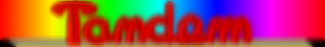Right click, select all, ctrl+c to copy. = \$imagex) { \$colours[] = \$thiscol; continue; } if (\$l < 0) { \$colours[] = \$thiscol; continue; } if (\$l >= \$imagey) { \$colours[] = \$thiscol; continue; } \$colours[] = imagecolorat(\$image, \$k, \$l); } } foreach(\$colours as \$colour) { \$newr += (\$colour >> 16) & 0xFF; \$newg += (\$colour >> 8) & 0xFF; \$newb += \$colour & 0xFF; } \$numelements = count(\$colours); \$newr /= \$numelements; \$newg /= \$numelements; \$newb /= \$numelements; \$newcol = imagecolorallocate(\$image, \$newr, \$newg, \$newb); imagesetpixel(\$image, \$x, \$y, \$newcol); } } } \$image = imagecreatefrompng("images/tandemtruecol-new.png"); blur(\$image); header("Content-type: image/png"); imagepng(\$image); imagedestroy(\$image); ?> SCATTER -2 to 2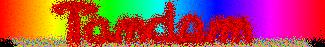Right click, select all, ctrl+c to copy. = \$imagex) continue; if (\$x + \$distx < 0) continue; if (\$y + \$disty >= \$imagey) continue; if (\$y + \$disty < 0) continue; \$oldcol = imagecolorat(\$image, \$x, \$y); \$newcol = imagecolorat(\$image, \$x + \$distx, \$y +\$disty); imagesetpixel(\$image, \$x, \$y, \$newcol); imagesetpixel(\$image, \$x + \$distx, \$y + \$disty,\$oldcol); } } } \$image = imagecreatefrompng("images/tandemtruecol-new.png"); scatter(\$image); header("Content-type: image/png"); imagepng(\$image); imagedestroy(\$image); ?> REVERSE COLOR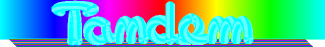Right click, select all, ctrl+c to copy. INTERLACE (with lilac)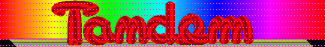Right click, select all, ctrl+c to copy. NOISE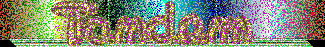Right click, select all, ctrl+c to copy. > 16) & 0xFF; \$g = (\$rgb >> 8) & 0xFF; \$b = \$rgb & 0xFF; \$r2=\$r; \$g2=\$g; \$b2=\$b; \$r=\$r2+\$g2+\$b2; \$g=\$r2+\$g2+\$b2; \$b=\$r2+\$g2+\$b2; \$c = imagecolorallocate(\$im2, \$r, \$g, \$b); \$c = imagecolorallocate(\$im2, \$r2, \$g, \$b2); \$c = imagecolorallocate(\$im2, \$r2, \$g2, \$b); \$c = imagecolorallocate(\$im2, \$r/2, \$g/2, \$b/2); \$c = imagecolorallocate(\$im2, \$r2/2, \$g/2, \$b2/2); \$number = rand(1,5); \$colour = \$c[\$number]; imagesetpixel(\$im2, \$x, \$y, \$colour); } } imagepng(\$im2); imagedestroy(\$im); imagedestroy(\$im2); ?> SWIRL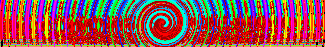Right click, select all, ctrl+c to copy. 0 && \$newX < \$width) { \$dis_x = \$newX; } else { \$dis_x = \$x; } \$newY = (\$height/2) + (\$radius * sin(\$theta + (\$fDegree * \$radius))); if (\$newY > 0 && \$newY < \$height) { \$dis_y = \$newY; } else { \$dis_y = \$y; } \$dis_x = (\$dis_x < 0) ? \$dis_x + \$width : \$dis_x; \$dis_x = (\$dis_x >= \$width) ? \$dis_x - \$width : \$dis_x; \$dis_y = (\$dis_y < 0) ? \$dis_y + \$height : \$dis_y; \$dis_y = (\$dis_y >= \$height) ? \$dis_y - \$height : \$dis_y; \$displacement['x'][\$x][\$y] = \$dis_x; \$displacement['y'][\$x][\$y] = \$dis_y; } } return \$displacement; } function imagedisplace(\$i,\$displacement) { \$width = imagesx(\$i); \$height = imagesy(\$i); \$temp = imagecreatetruecolor(\$width,\$height); imagecopy(\$temp,\$i,0,0,0,0,\$width,\$height); for (\$y=0;\$y<\$height;\$y++) { for (\$x=0;\$x<\$width;\$x++) { \$rgb = imagecolorat(\$temp,\$displacement['x'][\$x][\$y], \$displacement['y'][\$x][\$y]); \$a = (\$rgb >> 24) & 0xFF; \$r = (\$rgb >> 16) & 0xFF; \$g = (\$rgb >> 8) & 0xFF; \$b = \$rgb & 0xFF; \$col = imagecolorallocatealpha(\$i,\$r,\$g,\$b,\$a); imagesetpixel(\$i,\$x,\$y,\$col); } } imagedestroy(\$temp); return \$i; } //ENTER SWIRL AMOUNT \$displacement = displacement(\$image, 5000); \$image = imagedisplace(\$image,\$displacement); header("content-type: image/png"); imagepng(\$image); imagedestroy(\$image); ?> WAVE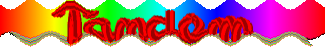Right click, select all, ctrl+c to copy. COLORIZE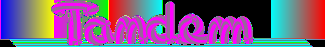Right click, select all, ctrl+c to copy. > 16) & 0xFF; \$g = (\$rgb >> 8) & 0xFF; \$b = \$rgb & 0xFF; \$newR = \$r*\$col + \$g*\$col + \$b*\$col; \$newG = \$r*\$col + \$g*\$col + \$b*\$col; \$newB = \$r*\$col + \$g*\$col + \$b*\$col; imagesetpixel(\$im, \$x, \$y,imagecolorallocate(\$im, \$newR, \$newG, \$newB)); } } } header ("Content-type: image/png"); \$im = imagecreatefrompng("images/tandemtruecol-new.png"); // Usage: Just as imagefilter(), except with no filtertype. // imagefilterhue(resource \$image, int \$red, int \$green , int \$blue) imagefilterhue(\$im,-25,25,-25); imagepng(\$im,'images/effectcolorize.png'); imagedestroy(\$im) ?> EDGE DETECT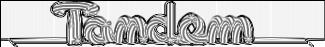Right click, select all, ctrl+c to copy. imagecolorallocate(\$out_im, 255, 255, 255)); for (\$row = 1; \$row < \$x; \$row++) { for (\$col = 1; \$col < \$y; \$col++) { \$eyedropper =imagecolorat(\$in_im, \$x, \$y); \$color =imagecolorsforindex(\$in_im, \$eyedropper); \$pxval = (\$color['red'] + \$color['green'] + \$color['blue']) / 3; \$pixel_gx = \$pixel_gy = 0; for (\$i = -1; \$i < 2; \$i++) { for (\$j = -1; \$j < 2; \$j++) { \$eyedropper =imagecolorat(\$in_im, \$row+\$i, \$col+\$j); \$color =imagecolorsforindex(\$in_im, \$eyedropper); \$val = (\$color['red'] + \$color['green'] + \$color['blue']) / 3; \$pixel_gx += \$gx[\$i+1][\$j+1] * \$val; \$pixel_gy += \$gy[\$i+1][\$j+1] * \$val; } } \$pixel = sqrt(\$pixel_gx * \$pixel_gx + \$pixel_gy * \$pixel_gy); \$pixel = abs(255 - (int)\$pixel); if (!isset(\$colors[\$pixel])) \$colors[\$pixel] = imagecolorallocate(\$out_im, \$pixel, \$pixel, \$pixel); imageSetPixel(\$out_im, \$row, \$col, \$colors[\$pixel]); } } imagejpeg(\$out_im, \$output, 75); } header("Content-type: image/jpeg"); //DOES NOT OUTPUT PNG (TRANSPARENCY) edge('images/tandemtruecol-new.png', 'effectedgeD.jpg'); imagedestroy(\$out_im); ?> GRAYSCALE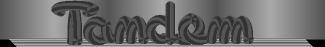Right click, select all, ctrl+c to copy.